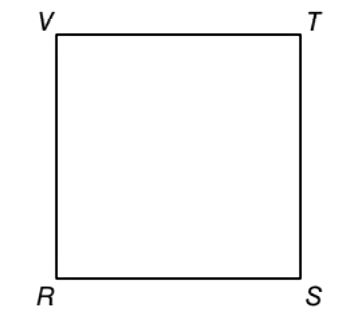Chapter 1.2, Problem 22E### Elementary Geometry for College St...

6th Edition
Daniel C. Alexander + 1 other
ISBN: 9781285195698

#### Solutions

Chapter
Section### Elementary Geometry for College St...

6th Edition
Daniel C. Alexander + 1 other
ISBN: 9781285195698
Textbook Problem
27 views

# Square R S T V has diagonals R T ¯ and S V ¯ (not shown). If the diagonals are drawn, how will their lengths compare? Do the diagonals of a square appear to be perpendicular?To determine

To find:

To compare the lengths of the diagonal of the square RSTV and to see whether the diagonals appears to be perpendicular.

Explanation

Calculation:

Given,

A square RSTV

The length of the diagonal of a square are always equal in measure.

Thus, the diagonals RT¯ and

### Still sussing out bartleby?

Check out a sample textbook solution.

See a sample solution

#### The Solution to Your Study Problems

Bartleby provides explanations to thousands of textbook problems written by our experts, many with advanced degrees!

Get Started